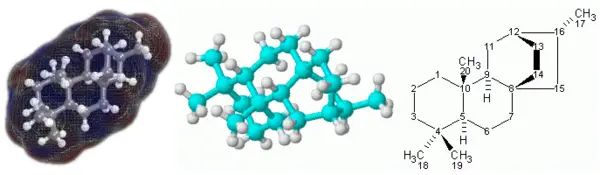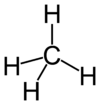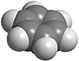# Molecule3D (left and center) and 2D (right) representations of the terpenoid molecule atisane.

In chemistry, a molecule is an entity comprising two or more atoms held together by natural forces in a definite spatial arrangement. The atoms are commonly joined together by shared pairs of electrons forming chemical bonds. Generally, each molecule is relatively stable and electrically neutral. In addition, a molecule is considered the smallest particle of a pure substance that retains its chemical composition and properties. While atoms are the original chemical building blocks of matter, the much, much more numerous stable molecules additionally serve as the building blocks of substances. The structures and functions of substances can be attributed to the physical and chemical interactions of their atoms and molecules.

## History

The concept of molecules was first introduced in 1811 by Amadeo Avogadro and was accepted by many chemists based on Dalton's laws of Definite and Multiple Proportions (1803-1808). On the other hand, most members of the physics community, with some notable exceptions (Ludwig Boltzmann, James Clerk Maxwell, and Willard Gibbs), thought of molecules as no more than convenient mathematical constructs, until the work of Jean Perrin in 1911. Philosophers such as Ernst Mach in the school of logical positivism also strenuously resisted the idea that molecules could really exist.

## Molecular chemistry and physics

The science of molecules is called molecular chemistry or molecular physics, depending on the focus. In theory, molecular chemistry deals with the laws governing interactions between molecules, resulting in the formation and breakage of chemical bonds; and molecular physics deals with the laws governing the structures and physical properties of molecules. In practice, however, this distinction is vague.In a molecule of methane, each hydrogen atom forms a covalent bond with the central carbon atom. Each covalent bond, represented as a line, is thought to involve the sharing of a pair of electrons.

A molecule may be composed of atoms of a single element or two or more elements joined in a fixed ratio. In either case, the atoms are bound together by shared pairs of electrons forming "covalent bonds." For this reason, the term molecular is sometimes used as a synonym for covalent.

The sharing of electrons is described by two theories, known as valence bond theory and molecular orbital theory, which are based on quantum mechanics. The electrons (conceived as "electron clouds," not discrete particles) are said to occupy certain energy levels ("orbitals") within the molecule. Although it is not always mentioned, it needs to be remembered that the positively charged nuclei in the atoms of a molecule play an essential role in holding the negatively charged electrons in their respective orbitals. Thus, both the atomic nuclei and the electrons are intimately involved in building each molecule.

If a molecule consists of two atoms, it is described as diatomic, and if it contains more than two atoms, it is called polyatomic. A number of diatomic molecules are composed of two atoms of the same element. Familiar examples are oxygen (O2), nitrogen (N2), hydrogen (H2), fluorine (F2), chlorine (Cl2), and bromine (Br2). Other diatomic molecules, such as carbon monoxide (CO), consist of atoms of two different elements. Examples of polyatomic molecules are water (H2O), ammonia (NH3), and carbon dioxide (CO2).

The noble gases (such as helium, neon, and argon) exist as single atoms. For the kinetic theory of gases, these atoms are regarded as "monatomic molecules."

Unlike molecular covalent compounds, ionic compounds do not yield well-defined "smallest particles" that would be consistent with the above definition of a molecule. In addition, no typical "smallest particle" can be defined for covalent crystals, which consist of repeating "unit cells" that extend indefinitely. For instance, in graphite, the unit cells extend to form planar sheets, and in diamond, the unit cells extend in three dimensions.

The term unstable molecule is used for a very reactive, short-lived species, such as a "radical" or "molecular ion."

### Size

Most molecules are too small to be seen with the naked eye, but their images can be observed by the technique of electron microscopy. The smallest molecule is that of hydrogen (H2). In this case, the distance between the two atoms is 0.15 nanometers (1.5 Å), but the size of its electron cloud is difficult to define precisely. Under standard conditions (25° C, 100 kPa), molecules range in size from a few to several dozen Å. Large molecules—such as DNA, RNA, and proteins—are described as macromolecules.

### Polarity

In general, a molecule as a whole is electrically neutral. There may, however, be an uneven distribution of electrical charge within the molecule, causing it to be electrically polar. This phenomenon is attributed to a property called electronegativity. In the bond between two atoms of different elements, the atom with higher electronegativity pulls negatively charged electrons closer to itself, creating a negative charge around itself and leaving a positive charge on the other atom. As a result of this type of uneven charge distribution, the molecule becomes polar.

## Chemical formulas

Main article: Chemical formula

In chemistry, the composition of a substance is expressed by its chemical formula. There are two types of chemical formulas: molecular formula and empirical formula.

The molecular formula of a compound reflects the exact number and types of atoms that make up each molecule of the compound, using the chemical symbols for the elements. If the molecule contains two or more atoms of a given element, the number of atoms is indicated as a subscript after the symbol for that element. For example, the molecular formula for water is H2O, indicating that each molecule contains two hydrogen atoms and one oxygen atom.

The molecular formula of a substance can be used to calculate the molecular mass—that is, the mass of each molecule of the substance. The molecular mass is the sum of masses of all the atoms in the molecule.

The empirical formula of a chemical is a simple expression of the relative number of each type of atom in each molecule of the substance. The numbers of atoms are expressed in their simplest integer ratios. For example, acetylene has the molecular formula C2H2, but its empirical formula is CH. Glucose has the molecular formula C6H12O6, but its empirical formula is CH2O. In some cases, the empirical formula is the same as the molecular formula. Examples include water (H2O) and methane (CH4).

Empirical formulas are the standard way to represent ionic compounds, such as CaCl2, and covalent networks, such as SiO2. The term "empirical" refers to the use of an experimental method to determine the relative percentages of each element in a pure chemical substance.

## Molecular geometry

Molecular geometry, or molecular structure, is the three-dimensional arrangement of the atoms that constitute a molecule. Each molecule has a fixed "equilibrium geometry"—consisting of its bond lengths and angles—about which the atoms continuously oscillate through vibrational and rotational motions. The geometry can be inferred through spectroscopic studies of the compound, or predicted using the valence bond theory. The molecular geometry depends on several factors, such as how the atoms bond together and the types of chemical bonds. The molecule's properties, particularly its reactivity, is greatly determined by its molecular geometry.

Two (or more) substances may be made up of molecules that share the same molecular formula but with different molecular structures. Then those substances will have different properties and are called isomers. For example, n-butane and isobutane are structural isomers, each containing four carbon and ten hydrogen atoms. In n-butane, the four carbon atoms are arranged as a straight chain, while in isobutane, they are arranged as a branched chain.

## Molecular ModelsA space-filling model of a molecule of benzene.

To visualize how molecules look in three dimensions, chemists use molecular models. The models most often used are known as "ball-and-stick" and "space-filling" models, usually made of wood or plastic.

In the ball-and-stick model, balls of various colors are used to represent atoms of different elements. The balls are usually of the same size, except those that represent hydrogen atoms, which are smaller. Sticks (or springs) are used to represent chemical bonds between the atoms, and they also show the angles between the bonds (called "bond angles"). The sticks, however, often show chemical bonds with exaggerated lengths.

In space-filling models, truncated balls are used to represent atoms. The balls are attached to one another with snap fasteners, so no chemical bonds are visible. The sizes of the balls are proportional to the sizes of the atoms (atomic radii).

## Molecular spectroscopy

Main article: Spectroscopy

Molecular spectroscopy is a technology that is extremely useful for determining the structural features of molecules of a substance. A sample of the substance is exposed to a probing signal, which can be electromagnetic radiation or a beam of electrons or other elementary particles at a known energy level. The molecules may respond in various ways: (a) by absorbing the radiation (absorption spectroscopy); (b) by emitting another signal (emission spectroscopy); (c) by fragmentation; or (d) by chemical changes.

By analyzing the spectrum of energy that is absorbed or emitted by the molecules, or the fragments released or chemical changes produced, scientists can determine the composition and molecular structures of substances. To extract maximum information about a substance, spectroscopy is often coupled with chemical analyses and computations.